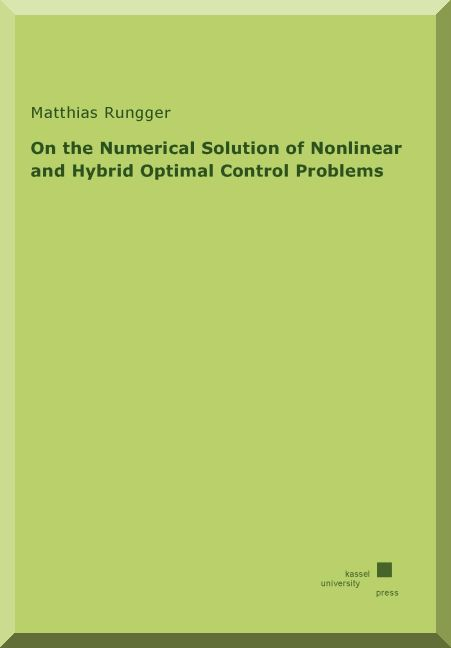On the Numerical Solution of Nonlinear and Hybrid Optimal Control Problems

Rungger, Matthias

kassel university press, ISBN: 978-3-86219-366-0, 2012, 148 Seiten

Zugl.: Kassel, Univ., Diss. 2011Inhalt: In this doctoral thesis an algorithm for the solution of a nonlinear and hybrid optimal control problem is developed and analyzed. The algorithm is based on the Dynamic Programming Principle, where the infinite problem is approximated by a finite problem. The finite approximation is obtained by a partitioning of the state space, together with a time and input space discretization. The resulting finite optimal problem is equivalent to a shortest path problem. Therefore, it is solvable by known graph theoretic methods. The outcome of this procedure is a function which is derived from the solution of the shortest path problem that associates to every partition element a control input. The control function is then applied to the control system in a sample-and-hold scheme, i.e., at each sample time the control input which is associated to the partition element that contains the current state, is applied to the system and kept constant over the sampling interval. It is shown that the performance of the resulting closed-loop system converges to the optimal performance as the discretization parameters decrease to zero. Additionally, based on an attainable set computation, the stability of the closed-loop system is ensured for all initial states within the partition elements for which the computation of the control input was successful.
The working principle of this algorithm is already known since the beginning of Dynamic Programming for nonlinear optimal control problems. In this thesis the described
approach is extended to a certain class of hybrid systems with autonomous and controlled transitions. Furthermore, a new algorithm which is based on Dijkstra’s shortest path
algorithm is developed. Unlike to known methods, the computation of the finite approximation and the computation of the solution of the shortest path problem are combined within one algorithm. That is, the most computational intensive part in the computation of the finite approximation, the attainable set computation, is executed at run-time of the shortest path algorithm, and thus is avoided, after a successful input for a partition element is found. As a result, the run-time and the memory complexity of the overall computation is reduced. For the considered numerical examples a reduction of up to 50% of the run-time and the memory demand of the computations has been observed.

Die Publikation ist in folgenden Formen erhältlich:

Volltext (pdf-Datei, nicht ausdruckbar - 1.20 MB)
Volltext (pdf-Datei, ausdruckbar, kostenpflichtig - 1.20 MB) 20.00 Euro
(kostenfrei im Netz der Universität Kassel - Im Netz der Uni Kassel befinden Sie sich, wenn Sie z.B. an einem Rechner im ITS, Ihrem Arbeitsplatz an der Uni oder auch in der Multimediathek der Bibliothek befinden.)